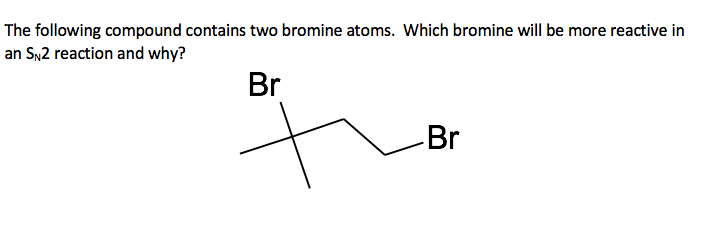# The following compound contains two bromine atoms. Which bromine will be more reactive in an SN2...

###### Question:The following compound contains two bromine atoms. Which bromine will be more reactive in an SN2 reaction and why? Br -Br

#### Similar Solved Questions

##### Consider the following trigonometric function: 2(tan(x)+3) = 5+tan(x) , 0 is less than or equal to...
Consider the following trigonometric function: 2(tan(x)+3) = 5+tan(x) , 0 is less than or equal to x and x < 2pi 1) use substitution to isolate tan(x) on a side by itself 2) Find all solutions to this equation...
##### Write the equilibrium‑constant expression for the reaction shown in terms of [A], [B], [C], and [D]....
Write the equilibrium‑constant expression for the reaction shown in terms of [A], [B], [C], and [D]. A(g)+B(g)−⇀↽−2C(g)+D(g) Note that Kc, which is sometimes symbolized as K or Keq, denotes that the equilibrium constant is expressed using molar concentrations. For this ...
##### Count 10 more numbers for the following bases started with the first given number: 1. Octal:...
Count 10 more numbers for the following bases started with the first given number: 1. Octal: 267 2. Base 3: 2102 3. Binary: 110101 4. Base 5: 3434...